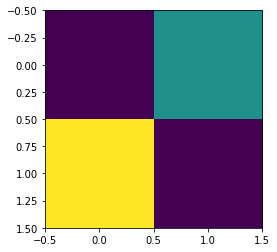In :
``1+1``
Out:
``2``
In :
``import numpy as np``
In :
``x = np.array([[1,2],[3,1]])``
In :
``x.shape``
Out:
``(2, 2)``
In :
``import matplotlib.pyplot as pp``
In :
``pp.imshow(x)``
Out:
``<matplotlib.image.AxesImage at 0x7f1154b24860>``In [ ]:
`` ``